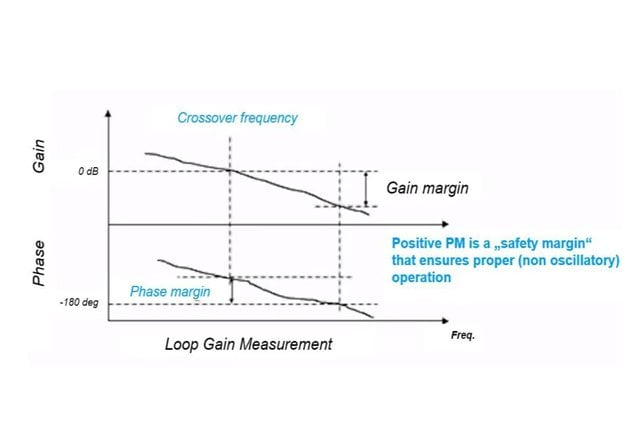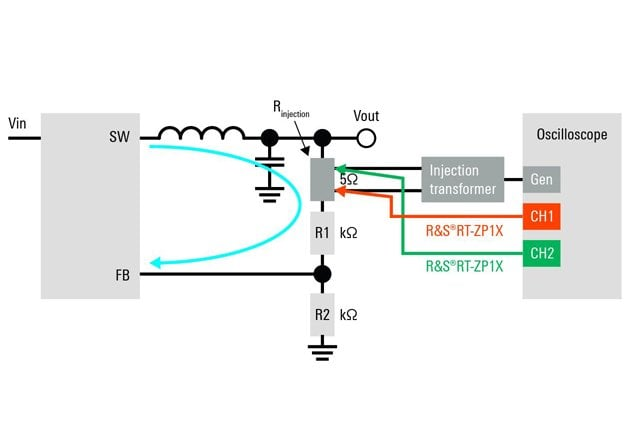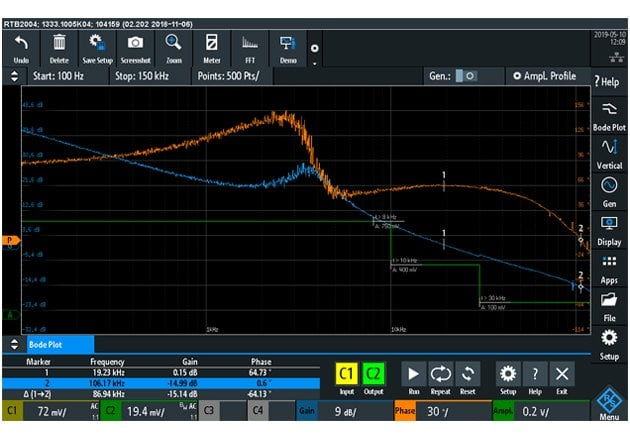Technical Article

# How to Analyze Control Loop Stability with Bode Plots Using Modern Oscilloscopes

January 21, 2020 by Markus Herdin

## This article presents important topics to consider in order to make accurate Bode plots for control loops in switched mode power supplies.

Traditionally, the method of choice has been to observe the step response and judge from the timing of whether the control loop is stable and fast enough. However, judging the level of stability from a step response is more an art than a science. Mere observation of the step response makes it difficult to assess how changes in design improve the performance of a control loop.

Stability also depends on the operation point of a power supply. A control loop might be stable for heavy loads but become nearly unstable for low loads. Consequently, the verification of different operational points is essential.

Control loops have been researched for decades. A reliable method for verification of stability is the closed-loop response (CLR) measurement, illustrated using a Bode plot graph (see below). In the past, measuring the closed-loop response required special equipment such as a vector network analyzer. The price of the equipment and the inconvenience of setting up an additional measurement instrument often prevented the engineers from using closed-loop response measurements.

Nowadays, modern oscilloscopes feature signal generators and flexible, powerful signal processing capabilities. This makes it possible to perform automatic closed-loop response measurements with the oscilloscope that an R&D engineer already has for everyday power supply tests. This capability is typically an optional software extension of the oscilloscopes, making it a cost-effective alternative to standalone solutions.

A Bode plot is a graph depicting the frequency response of a system. It combines a Bode magnitude plot that describes the magnitude of the frequency response in dB and a Bode phase plot that describes the phase shift.

With these two plots it is possible to measure whether a control loop is stable or unstable and how big the “safety” margin is. At the crossover frequency, where the gain of the control loop goes down to 0dB, the phase margin can be measured. It is the remaining phase to -180° phase shift, the point where the control loop would get unstable. The phase margin, therefore, measures the “safety” margin for the phase.

At the frequency where the phase of the control loop reaches-180°, the gain margin can be measured. The gain margin is the negative gain at this frequency, i.e. measuring the additional gain would be needed in order to reach the point of instability. Both phase margin, as well as gain margin, are therefore the safety margin for stability.##### Figure 1: Loop Gain Measurement

In the oscilloscope, the Bode plot measurement is based on the built-in waveform generator and two measurement channels. The waveform generator is used to generate a disturbance signal that is fed into the control loop and the two measurement channels are necessary to measure both the disturbance signal itself as well as the reaction of the control loop. The ratio between both is the (complex) control loop gain containing magnitude gain and phase. The oscilloscope automatically sweeps through all measurement frequency and plots the gain and phase responses.

This article presents the most important topics to consider in order to make accurate Bode plots for control loops in switched-mode power supplies.

### A) Selecting the Right Injection Point

To measure the control loop response, the loop has to be broken at a suitable point and a perturbation signal injected at this point. A simple method of performing this is to add in the feedback loop an additional resistor that is small enough not to influence the actual feedback loop significantly. Injecting a signal across the resistor makes it possible to insert a perturbation signal into the control loop and measure the response to this perturbation at the output of the power supply. This is called the voltage injection method. It is important to add the resistor at a point where the impedance in the direction of the feedback loop is much larger than the impedance looking backward. Figure 2 shows how to correctly carry it out.### B) Injection Transformer

The injection transformer is connected between the signal generator and the power supply converter in order to inject a small error signal into the control loop. The measurement concept requires a connection to the DUT, and it is important to ensure that the transformer will not change the behavior of the loop. Therefore, the transformer has to provide galvanic isolation (safety, instrument protection, signal integrity), inhibit DC voltages from the generator and have a relatively flat gain over the whole frequency range. When choosing a transformer, the permeability core and the parasitic capacitance between the primary and secondary side should also be observed.

### C) Amplitude Profiling

A control loop is by nature very sensitive to a disturbance signal as it has the basic task of steering the control so that the effects of the disturbance signals (i.e. error signals) are minimized. When injecting a disturbance signal into a control loop to measure their response, it is therefore important to carefully choose the right amplitude. Basically, the following rules apply:

• The injection signal amplitude must be low enough that it provides only a small signal perturbation. This is important to avoid overdriving the active components.
• The injection signal level must be high enough above the noise level to allow an accurate measurement result. As the gain of the control loop varies over frequency, so does the optimum injection signal level. This is what amplitude profiling does, i.e. allows the definition of the amplitude level of the injection signal dependent on the measurement frequency.### D) Probing

Key to good measurements with oscilloscopes is choosing the right probe. Probes differ in voltage levels, attenuation ratios, bandwidth and many other parameters. Depending on the application, different parameters are important.

Standard passive probes typically supplied with an oscilloscope have an attenuation factor of 10:1 and a bandwidth in the range of 500 MHz. While the bandwidth of such a probe is totally sufficient for control loop measurements, the additional attenuation is a significant disadvantage.

For control loop response measurements, sensitivity and therefore low-noise probing is most important. For that reason, 1:1 probes are usually the best choice, in particular since the measurement voltage is often not too high and required measurement bandwidths are in the range of only up to a few MHz.

When using power supplies with higher output voltages, high-voltage passive probes or high-voltage differential probes are recommended.

### E) Stability Measurements Overall Conditions

In switched-mode power supply circuits, changes in line, load, and temperature sometimes degrade phase margin markedly from the nominal value. A typical example is a low-load situation, where power supplies might go into discontinuous current conduction mode, with a different control loop characteristic than the characteristics of the control loop in standard load situations. In order to be sure that the power supply is stable in all scenarios, it is recommended to perform Bode plot measurements for all operation points.

### An All-in-one Solution

Oscilloscopes have become all-in-one solutions for many measurement problems electrical engineers face. Having the capability of performing Bode plots using the built-in waveform generator extends the capabilities of the oscilloscopes to yet another application that is particularly important for power supply design. This saves space on the engineer’s desk and eliminates the need for investing in a specialized testing solution for control loop analysis.